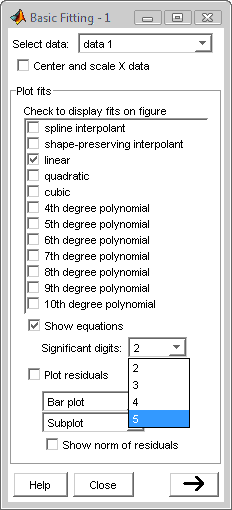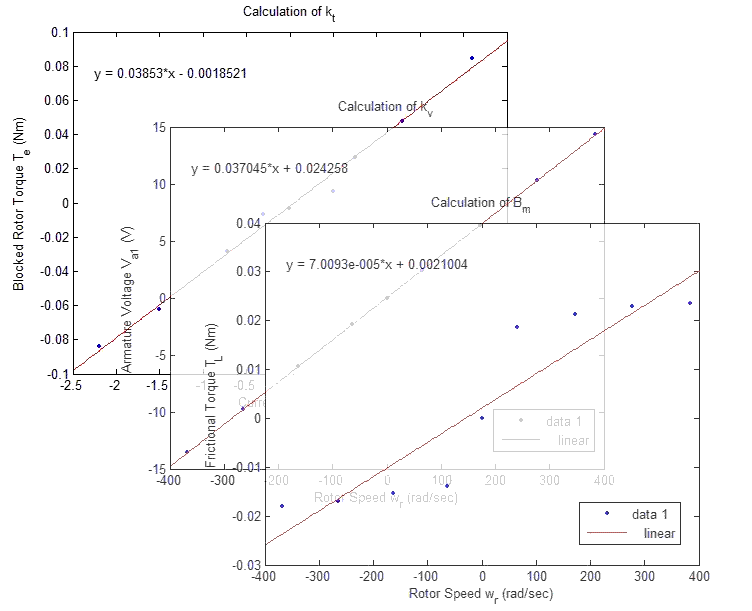# Lab 5a Tips: DC Motor

## Pre-lab

• Equation (1)-(3) in state space form will look like the system below. The vector u represents inputs to the system (va and TL).• For steady state use capital letters for variables (capital ω is Ω ).
• Steps for finding the transfer functions.
1. Start from equations (1)-(3).
2. Neglect LAA.
3. Combine equations (1)-(3) to eliminate ia.
4. Convert the resulting equation to the frequency domain through application of Laplace transforms using the identities below. Note that the capital form ω is Ω, the symbol we choose for ω in the frequency domain, and that it is safe to assume ωr(0) = 0.
• Linearity: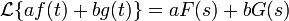• Differentiation: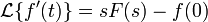5. Solve the resulting equation for Ω (s). Your result should be of the form given below where the parentheses represent constant terms.6. Solve the above equation for the transfer functions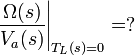.
• To Find ωr(t), use the first transfer function above and solve for ωr(t) given va(t) = Vau(t). In other words, solve the following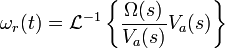.

## In-lab

• You can complete the entire lab with this one model.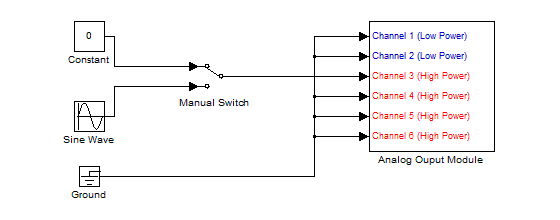• To convert from torque transducer voltage (as read on ocilliscope) to actual torque use the relations: 1 V = 15 oz-in and 1 N-m = 141.6 oz-in
• To find kt, kv, and Bm, use Matlab's built in data fitting features.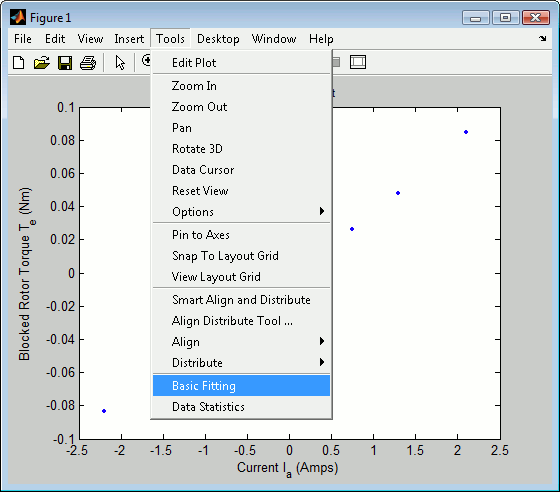• Select 'linear', 'show equations', and at least three significant digits.